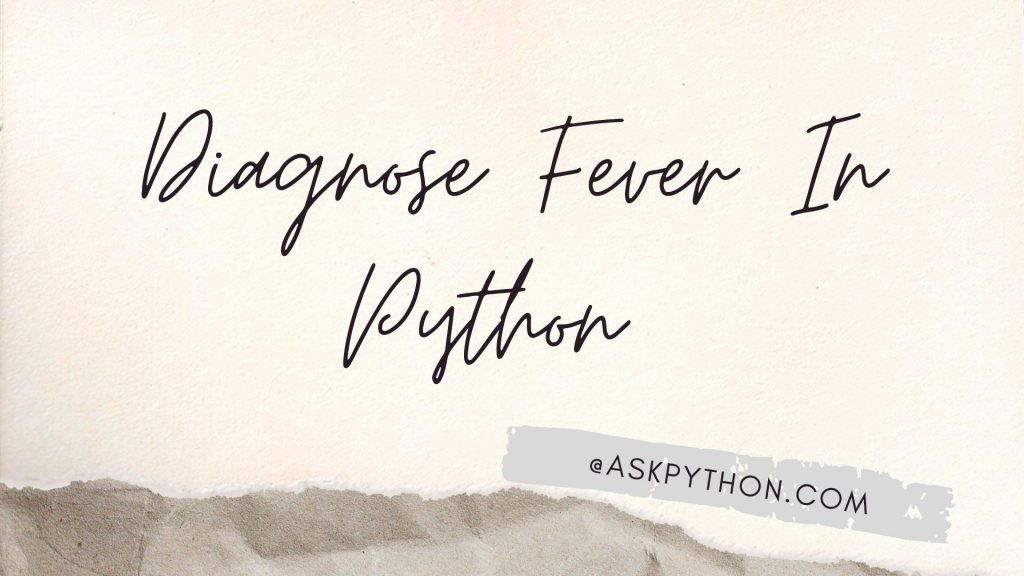# Diagnose Fever In Python [Easy CLI Method]Hey coder! In this tutorial, we will learn about one of the frequently asked Python programming questions, can you diagnose fever using the Python programming language?

A fever is a body temperature that is higher than normal. A normal temperature can vary from person to person, but it is usually around 98.6 °F (37 °C). A fever is not a disease. It is usually a sign that your body is trying to fight an illness or infection.

## Implementing Fever Detection in Python

We will start off by asking the user if they will be entering the temperature in Celcius or Fahrenheit. This can make a significant difference in the decision-making. Now we will check if the input is a C or an F or if there is a wrong input.

```temp = input("Would you like to enter your temperature in Celcius or Fahrenheit: ")
if temp.upper() == "C":
pass
elif temp.upper() == "F":
pass
else:
pass
```

Let’s go block after block to get the final code. The first block is when the temperature scale entered is ‘C’. In such a case the user can enter the temperature and if the temperature is greater or equal to 37.8 then the person has a fever. Otherwise, the person doesn’t have a fever. The temperature is converted to float for better diagnosis. Look at the code below.

```temp = input("Would you like to enter your temperature in Celcius or Fahrenheit: ")
if temp.upper() == "C":
result = input("Enter your body temprature in Celcuis: ")
r = float(result)
if r >= 37.8:
print("You've a fever")
else:
print("You don't have a fever")
elif temp.upper() == "F":
pass
else:
pass
```

The next block we have is when the input is ‘F’. In this case, the threshold temperature is 98.6. The rest remains the same as above. Take the input and convert the input to float for better analysis. Look at the code snippet below.

```temp = input("Would you like to enter your temperature in Celcius or Fahrenheit: ")
if temp.upper() == "C":
result = input("Enter your body temprature in Celcuis: ")
r = float(result)
if r >= 37.8:
print("You've a fever")
else:
print("You don't have a fever")
elif temp.upper() == "F":
result1 = input("Enter your body temprature in Fahrenheit:")
r1 = float(result1)
if r1 >= 98.6:
print("You've a fever")
else:
print("You don't have a fever")
else:
pass
```

The last block we have is when the user gives the wrong input. In such a case a simple statement is printed as the output. Look at the code below.

```temp = input("Would you like to enter your temperature in Celcius or Fahrenheit: ")
if temp.upper() == "C":
result = input("Enter your body temprature in Celcuis: ")
r = float(result)
if r >= 37.8:
print("You've a fever")
else:
print("You don't have a fever")
elif temp.upper() == "F":
result1 = input("Enter your body temprature in Fahrenheit:")
r1 = float(result1)
if r1 >= 98.6:
print("You've a fever")
else:
print("You don't have a fever")
else:
```

## The Complete Code for Fever Detection in Python

```temp = input("Would you like to enter your temperature in Celcius or Fahrenheit: ")
if temp.upper() == "C":
result = input("Enter your body temprature in Celcuis: ")
r = float(result)
if r >= 37.8:
print("You've a fever")
else:
print("You don't have a fever")
elif temp.upper() == "F":
result1 = input("Enter your body temprature in Fahrenheit:")
r1 = float(result1)
if r1 >= 98.6:
print("You've a fever")
else:
print("You don't have a fever")
else:
```

## Some Sample Outputs

```Would you like to enter your temperature in Celcius or Fahrenheit: C
Enter your body temprature in Celcuis: 100
You've a fever

Would you like to enter your temperature in Celcius or Fahrenheit: F
Enter your body temprature in Fahrenheit:56
You don't have a fever

Would you like to enter your temperature in Celcius or Fahrenheit: j